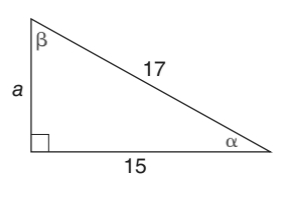Chapter 11.1, Problem 3EElementary Geometry For College St...

7th Edition
Alexander + 2 others
ISBN: 9781337614085

Solutions

Chapter
SectionElementary Geometry For College St...

7th Edition
Alexander + 2 others
ISBN: 9781337614085
Textbook Problem

In Exercises 1 to 6, find sin     α and sin     β for the triangle shown.To determine

To find:

The angle sinαandsinβ

Explanation

In a right angle triangle,

1) The side PQ, which is opposite to the right angle PRQ is called the hypotenuse.

(The hypotenuse is the longest side of the right triangle.)

2) The side RQ is called the adjacent side of angle θ.

3) The side PR is called the opposite side of angle θ.

Hence, the sine ratio for an acute angle is oppositehypotenuse

Given:

By using Pythagoras theorem, that is

c2=a2+b2

From, the diagram we have c=17andb=15

172=a2+152289=a2+225a

Still sussing out bartleby?

Check out a sample textbook solution.

See a sample solution

The Solution to Your Study Problems

Bartleby provides explanations to thousands of textbook problems written by our experts, many with advanced degrees!

Get Started

In Exercises 49-62, find the indicated limit, if it exists. 49. limx1x21x1

Applied Calculus for the Managerial, Life, and Social Sciences: A Brief Approach

56. Profit If the profit function for a product is given by Find .

Mathematical Applications for the Management, Life, and Social Sciences

Convert the expressions in Exercises 6584 to power form. 1(x2+1)334(x2+1)3

Finite Mathematics and Applied Calculus (MindTap Course List)

Evaluate the integral. 36. /4/2cot3xdx

Single Variable Calculus: Early Transcendentals

For 0

Study Guide for Stewart's Multivariable Calculus, 8th